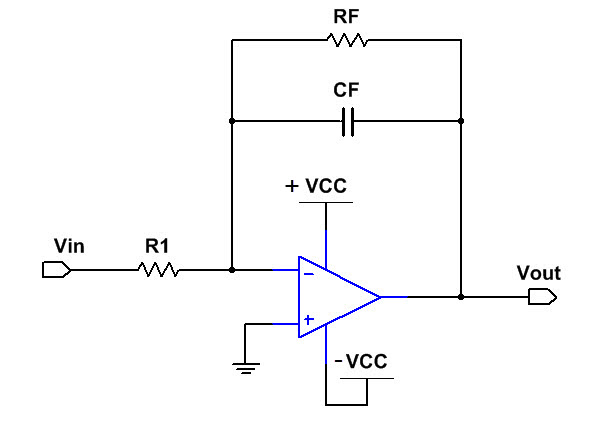# Practical Op-Amp Integrator Online Calculator

 Dual Supply Op-Amp Integrator Calculator(DC coupled) Inputs: Vin: V f: Hz KHz MHz VCC: V |A|: CF: µF nF pF Formula Used: $$R_{F}=\frac{1}{C_{F} f},$$$$\\$$ Theoritical Results: RF: R1: fa: fb: S:Single Supply Op-Amp Integrator Calculator(DC coupled) Inputs: Vin: V f: Hz KHz MHz VCC: V |A|: CF: µF nF pF Formula Used: $$R_{F}=\frac{1}{C_{F} f},$$$$\\$$ Theoritical Results: RF: R1: fa: fb: S:### About Op Amp Integrator Calculator

The above op-amp integrator calculator calculates the theoritical value of components required for input signal characteristics and op-amp characteristics. Op-amp integrator formula are provided. Op-Amp Integrator solved problem are as follows: